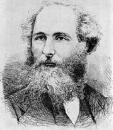Home

Lecture Notes

PHY 218: Electricity and Magnetism II
Prof. S. Teitel stte@pas.rochester.edu ---- Spring 2016

## Lecture Notes

The lecture note files correspond roughly to the material presented in a given day's lecture. But you may on occassion find the end of one day's lecture at the start of the file for the next day's lecture, so please look there if you think there might be something missing.

• Lecture 0 - A brief history of electromagnetism

• Lecture 1 - Review of electro- and magneto- statics, electromotive force, electromagnetic induction in a loop moving in a static magnetic field

• Lecture 2 - Magnetic induction continued, Faraday's Law, Lenz' Law, review of magnetostatics and Biot-Savart Law

• Lecture 3 - Levi-Civita tensor, mutual and self inductance, LR circuit

• Lecture 4 - Force between two current carrying loops and the energy stored in a magnetostatic current configuration

• Lecture 5 - Examples

• Lecture 6 - More examples, Maxwell's correction to Ampere's Law, conservation of energy in presence of electromagnetic fields

• Lecture 7 Electromagnetic energy density and energy current (Poynting vector) of electromagnetic fields

• Lecture 8 - Conservation of momentum, Maxwell stress tensor, electromagnetic momentum density and angular momentum density, magnetic monopoles, Maxwell's equations in potential form

• Lecture 9 - Maxwell's equations in potential form, gauge transformations, Coulomb gauge, Lorentz gauge, electromagnetic waves in a vacuum

• Lecture 10 - Solutions to the wave equation, plane waves, spherical waves, simple harmonic wave, Fourier transform

• Lecture 11 - General solution to the homogeneous wave equation, Green's function for the inhomogeneous wave equation, longitudinal, transverse, and circular polarization

• Lecture 12 - Electromagnetic waves in a vacuum, energy and momentum of EM waves in a vacuum, macroscopic Maxwell's equations in matter, wave in a linear material with constant permeability and permeativity

• Lecture 13 - Frequency dependent polarizability, electric susceptibility and permittivity, non-local in time relation between displacement field D and electric field E

• Lecture 14 - Waves in a dielectric, dispersion relation, effects of complex permittivity, phase velocity, group velocity, normal and anomalous dispersion

• Lecture 15 - Wave pulse spreading, real and imaginary parts of the permittivity, real and imaginary parts of the wavenumber, regions of transparent propagation, resonant absorption, and total reflection

• Lecture 16 - Waves in a conductor: free current density and free charge density, frequency dependent conductivity, dispersion relation, good and poor conductors, skin depth

• Lecture 17 - Plasma frequency, longitudinal modes, reflection and transmission of waves at an interface

• Lecture 18 - Reflection and transmission continued: Snell's law, critical angle, total internal reflection, corrections to Snell's law for a dissipative medium, transmission into a highly absorptive medium

• Lecture 19 - Reflected and transmitted field amplitudes, coefficient of reflection, region of total reflection, reflection between two transparent media, Brewster's angle, Green's function for the wave equation

• Lecture 20 - Green's function for the wave equation, radiation from a localized oscillating charge density, expansion for the vector potential

• Lecture 21 - Electric dipole, magnetic dipole and electric quadrapole terms for radiation, magnetic fields in the electric dipole approximation, radiation zone
Review of magnetic dipole approximation in magnetostactics

• Lecture 22 - Electirc field, Poynting vector and radiated power in the electric dipole approximation, why is the sky blue?, magnetic dipole radiation

• Lecture 23 - Radiation from an arbitrary time-dependent charge distribution, Larmor's formula for the radiated power of an accelerated charge, radiation-reaction force, radiative decay of a classical atom

• Lecture 24 - Lienard-Wiechert potentials for a moving point charge, potentials and fields for a point charge moving with constant velocity

• Lecture 25 - Special relativity, Lorentz transformation, time dilation, FitzGerald contraction, simultaneity of events, proper time, proper length

• Lecture 26 - 4-vectors, Lorentz transformation matrix, 4-differential, proper time interval, 4-velocity, 4-acceleration, 4-gradient, wave equation operator, 4-current, 4-potential, the field strength tensor, transformation law for E and B fields

• Lecture 27 -Maxwell's equations in relativistic form, 4-momentum, Minkowski force, relativisitc kinetic energy, conservation of energy and momentum, the Lorentz force in relativistic form

• Lecture 28 - The relativisitic generalization of Larmor's formula